Faraday effect describes such a phenomenon:

If a magnetic field is applied to a medium in a direction parallel with light’s propagation direction, a rotation of the light’s polarization direction happens. This is best shown in a picture.The essence of Faraday effect or Faraday rotation is that it shows the interaction between light and a magnetic field in a medium.

In 1845 Faraday discovered that a magnetic field in the direction of propagation of a light beam in a transparent medium produces the effects of circular birefringence. Thus he established for the first time a direct connection between optics and electromagnetism - a connection he had long suspected to exist and for which he had searched for many years.

However, a half century passed before an explanation of the Faraday effect was formulated in terms of Maxwell's electromagnetic theory of light (developed in the 1860's) and the concept of the atomicity of charge which had evolved during the 19th century under the influence of electrochemistry and been validated by Thomson's discovery of the electron in 1897.

A Setup to Observe the Faraday Effect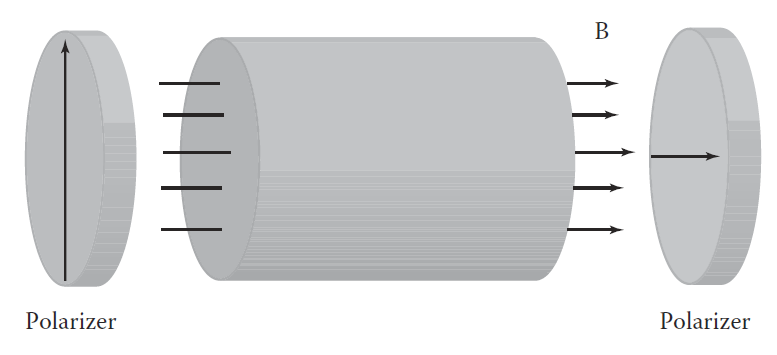In this setup, the magnetic field is set up so that the field lines are along the direction of the optical beam propagation. A linear polarizer allow light of one polarization into the cell. A second linear polarizer is used to analyze the result.

The magnitude of the rotation due to a field, H, over a path length, L is given by: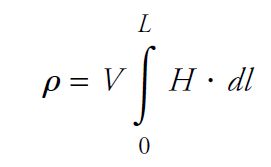where V is a constant known as the Verdet constant: V is a constant for any given material, but is wavelength and temperature dependent.

So if H is constant over the optical path, we then get:We can give a qualitative explanation for Faraday effect. Take a look at the picture below.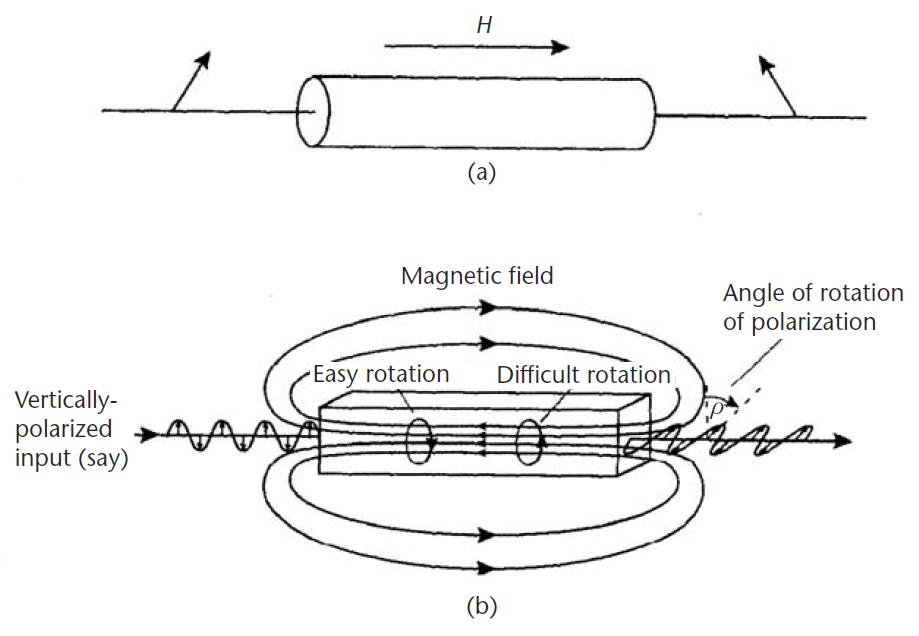When a magnetic field is applied to a medium, the electrons in the medium find it easier to rotate in one direction around the magnetic field than in the other.

The Lorentz force acts on a moving charge in a magnetic field, and this will act radially on the electron as it circles the field. The force will be outward for one direction of rotation and inward for the other.

The consequent electron displacement will lead to two different radii of rotation and thus two different rotational frequencies; and electric permittivities (ε). Hence the field will result in two different refractive indices, and thus to circular birefringence.

Light which is circularly polarized in the “easy” direction will travel faster than that polarized in the “hard” direction, leading to the observed effect.

The Nonreciprocal Nature of Faraday Effect

Nonreciprocal means that linearly polarized light is always rotated in the same ABSOLUTE direction in space, independently of the direction of propagation of the light.

A positive Verdet constant corresponds to L-rotation (anticlockwise) when the direction of propagation is parallel to the magnetic field and to R-rotation (clockwise) when the direction of propagation is anti-parallel. Thus, if a ray of light is passed through a material and reflected back through it, the rotation doubles.

This is best illustrated in the following picture.This is because in the Faraday magneto-optic effect, the rotation always takes place in the same direction with respect to the magnetic field (not the propagation direction) since it is this which determines easy and hard directions.

Hence, an observer always looking in the direction of light propagation will see different directions of rotation since he is, in one case, looking along the field and, in the other, against it.

Unlike the electro-optic effect, the magneto-optic effect causes a true rotation of the plane of polarisation for any input polarisation angle. In a simple electro-optic device, only pure rotations of 90° are available; all other intermediate voltages produce different degrees of elliptical polarisation states from a linear input state. A Faraday rotator however will truly rotate the plane of input polarisation through any angle (providing you can provide a strong enough magnetic field!

The Reciprocal Nature of Optically Active Crystal Polarization Rotation (i.e. waveplates)

On the other hand, the polarization rotation induced by optically active crystals, such as waveplates, is reciprocal.

If the polarization direction is rotated from right to left (say) on forward passage (as viewed by a FIXED observer) it will be rotated from left to right on backward passage (as viewed by the same observer).

So that back-reflection of light through an optically active crystal will result in light with zero final rotation, the two rotations having cancelled out. This is best shown in the following picture.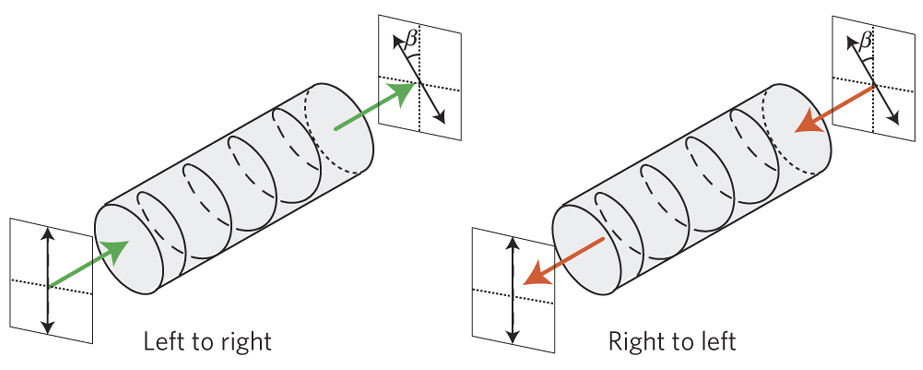This is because that the polarization rotation happened here is a result of a longitudinal spirality (represented as a helix) in the crystal structure. Hence, a rotation following the handedness of the spiral in the forward direction will be opposed by the spiral in the backward direction.

Magnetic Circular Birefringence - Becquerel’s Theory of the Faraday Effect

Experiments established early on that the Faraday rotation angle φ is proportional to the product of D(material length) and the magnetic field B. So we get

Φ = VDB

where V is a proportionality factor called the Verdet constant. However, how do we calculate the value V from the fundamental physics of light, magnetism, and matter?

The first step in understanding the Faraday effect in terms of electromagnetic theory and the atomic structure of matter was taken by H. Becquerel, in 1897.

Becquerel’s theory was based on the concept of the then newly discovered electron, but it didn’t include the idea of quantized energy states introduced by Planck in 1900, or the concept of light quanta, developed by Einstein in 1905.The particle, with mass m and charge q, is fixed to the end of a spring, with spring constant k, attached rigidly to the x axis. The directions of light propagation and the B field are parallel to the x axis; the E field is perpendicular to the x axis and rotates in the yz plane.

Here is the formula for Verdet constant derived by H. Becquerel: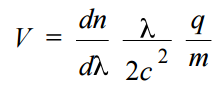where λ =2πc/ω is the vacuum wavelength of the light, m is mass, q is charge

Even though the Becquerel theory does not make use of quantum principles, it does provide a simple conceptual framework for a preliminary understanding of Faraday effect, and gives quantitative predictions for the Verdet constant which is remarkably close to the measured values.

The verdet constant for most materials is extremely small and is wavelength dependent. The effect is at its strongest in those substances containing paramagnetic ions such as terbium.

The highest Verdet constants are in fact found in terbium doped dense flint glasses and better still in crystals of terbium gallium garnet (TGG). Although expensive, this material has significant benefits over glasses and other substrates, notably excellent transparency, high optical quality and very high resistance to laser damage.

Although the Faraday effect is not itself chromatic (wavelength dependent), the verdet constant itself is quite strongly a function of wavelength. At 632.8nm, the verdet constant for TGG is reported to be -134 radT-1m-1 whereas at 1064nm, it has fallen to -40radT-1m-1. This behaviour means that the devices manufactured with a certain degree of rotation at one wavelength, will produce much less rotation at longer wavelengths.

The most common application for a Faraday rotator is when coupled with input and output polarisers to form an isolator.

The rotation is then set at 45°. The key property of a Faraday rotator which distinguishes it from say a quartz rotation plate, is that the effect is uni-directional.

A device which produces a clockwise rotation of the optical beam's polarisation axis through 45° when the beam propagates in one direction, will not reverse this effect for a beam passing back in the opposite direction, will produce an additional rotation of 45° for the reverse beam. This will then be at 90° to the input polarisation axis.

This is demonstrated in the illustration below:In the form of isolator shown, the rejected beams is not used and is simply discarded (in most applications it is not necessary to use a polariser with a side exit face, scattering of the rejected beam is sufficient).

This would for example be suitable when only rejection of back-reflected radiation is required. One could however make use of these beams to form an optical circulator by the addition of a few extra components.

The commonest use of Faraday isolators is in preventing laser oscillators from being damaged by strong back reflections from subsequent optics and especially from certain types of amplifiers where a significantly enhanced back reflection may occur.

They are also useful in preventing unwanted feedback from reducing the stability of line narrowed single frequency sources through mode pulling.

Rotators are also used for example in ring laser systems to introduce a loss mechanism (in conjunction with some other intra-cavity polarisation selective element) which is greater for one direction of propagation than for the other. This ensures uni-directional response. Usually such devices are not required to work at such high rotation angles as the 45° found in isolators so may be made much smaller.